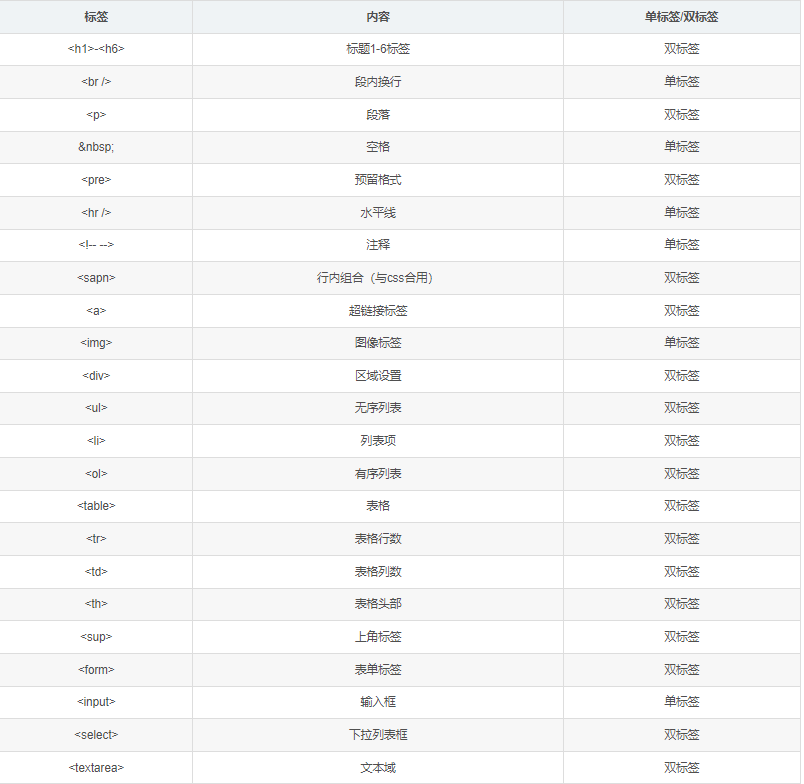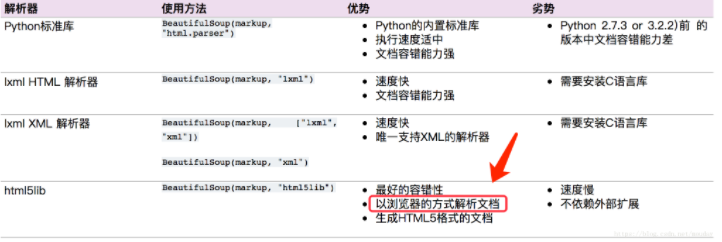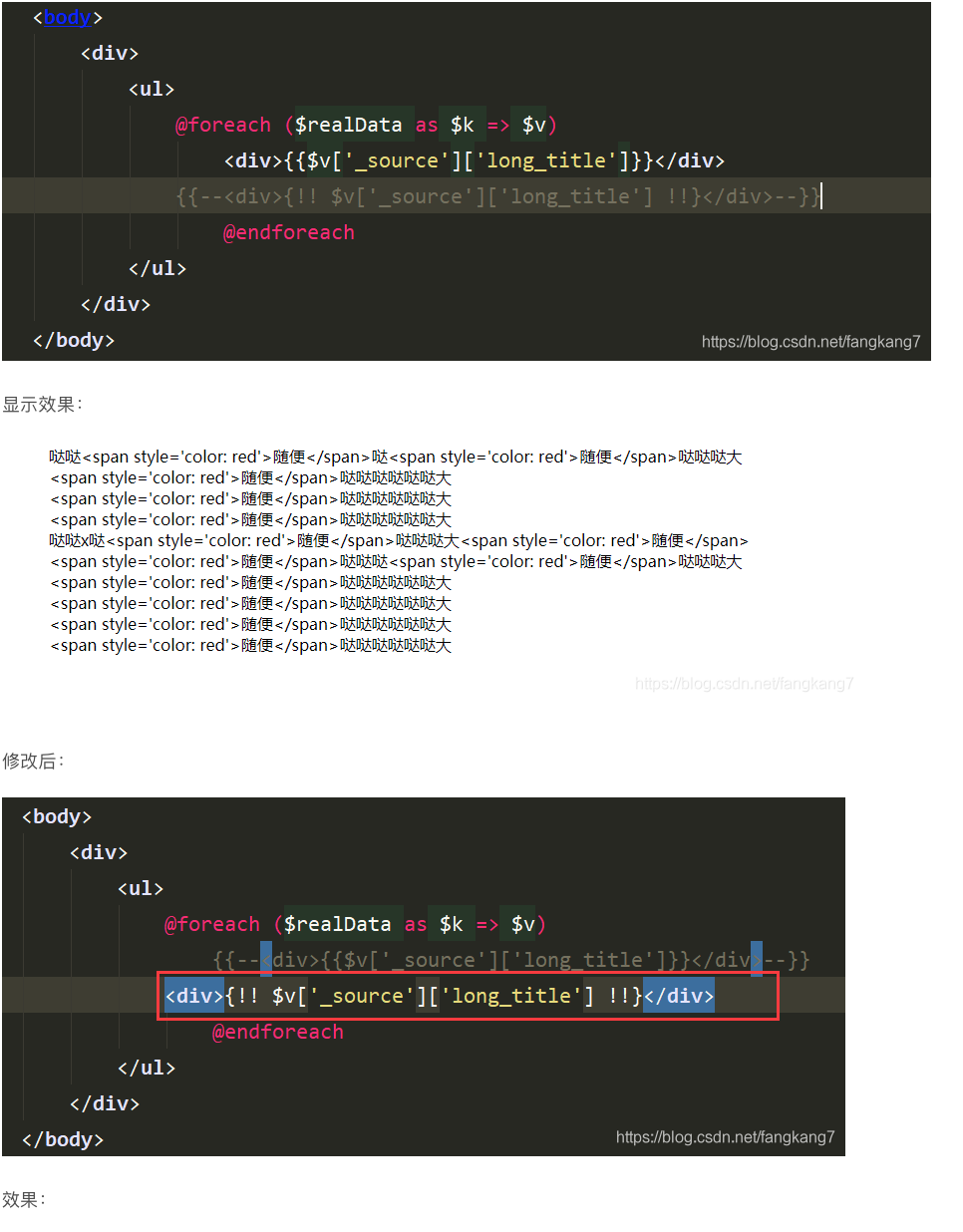+購廣写偬臥心

# 聞喘xpath糞孖document.querySelector劔塀僉夲匂序佩html盾裂↙屈⇄⦿制婢匯和xpath參宴屶隔屎夸

-----------------------------------------------------------------

-----------------------------------------------------------------

-----------------------------------------------------------------



 
 
 
 
 
 public class HtmlObject
 
 
 
 
 
 
 {
 
 
 
 
 
 
 private string _html = string.Empty;
 
 
 
 
 
 
 private List<string> _tags = new List<string>();
 
 
 
 
 
 
 private List<string> _self = new List<string>();
 
 
 
 
 
 
 private XmlDocument _xml = null;
 
 
 
 
 
 
 public string Html
 
 
 
 
 
 
 {
 
 
 
 
 
 
 get
 
 
 
 
 
 
 {
 
 
 
 
 
 
 return _html;
 
 
 
 
 
 
 }
 
 
 
 
 
 
 }
 
 
 
 
 
 
 public XmlDocument Xml
 
 
 
 
 
 
 {
 
 
 
 
 
 
 get
 
 
 
 
 
 
 {
 
 
 
 
 
 
 return _xml;
 
 
 
 
 
 
 }
 
 
 
 
 
 
 }
 
 
 
 
 
 
 public HtmlObject()
 
 
 
 
 
 
 {
 
 
 
 
 
 
 InitDefine();
 
 
 
 
 
 
 }
 
 
 
 
 
 
 public HtmlObject(string html)
 
 
 
 
 
 
 {
 
 
 
 
 
 
 _html = html;
 
 
 
 
 
 
 InitDefine();
 
 
 
 
 
 
 InitHtml();
 
 
 
 
 
 
 }
 
 
 
 
 
 
 public void Load(string file)
 
 
 
 
 
 
 {
 
 
 
 
 
 
 LoadHtml(FileHelper.FileToString(file));
 
 
 
 
 
 
 }
 
 
 
 
 
 
 public void LoadHtml(string html)
 
 
 
 
 
 
 {
 
 
 
 
 
 
 _html = html;
 
 
 
 
 
 
 InitHtml();
 
 
 
 
 
 
 }
 
 
 
 
 
 
 public void LoadUrl(string url)
 
 
 
 
 
 
 {
 
 
 
 
 
 
 Ajax ajax = new Ajax();
 
 
 
 
 
 
 ajax.AppendCss = false;
 
 
 
 
 
 
 ajax.AddFullPath = true;
 
 
 
 
 
 
 ajax.AutoSave = false;
 
 
 
 
 
 
 ajax.AutoUpdate = true;
 
 
 
 
 
 
 LoadHtml(ajax.Http(url));
 
 
 
 
 
 
 }
 
 
 
 
 
 
 private void InitDefine()
 
 
 
 
 
 
 {
 
 
 
 
 
 
 // 蕗苧徭液栽炎禰
 
 
 
 
 
 
 _self.AddRange(new string[] { "img", "br", "hr", "base", "meta", "link", "area" });
 
 
 
 
 
 
 }
 
 
 
 
 
 
 private void InitHtml()
 
 
 
 
 
 
 {
 
 
 
 
 
 
 _tags = new List<string>();
 
 
 
 
 
 
 XmlDocument xml = new XmlDocument();
 
 
 
 
 
 
 xml.LoadXml("<r />");
 
 
 
 
 
 
 MatchCollection mc = Regex.Matches(_html, @"<!(?!-)(?:[^<>'""]|(['""])[^'""]*\1)*?>|<([%\?])[\s\S]*?\2>|<!--[\s\S]*?-->|<(script|style)(?!\w)[^<>]*?>(?:[^'""]|(['""])[^'""]*\4)*?</\3(?!\w)[^<>]*?>|<(?![!%\?])(?:[^<>'""]|(['""])[^'""]*\5)*?>|[^<]+(?=<|$)", RegexOptions.IgnoreCase);              XmlNode node = xml.DocumentElement;              for (int i = 0; i < mc.Count; i++)              {              ParseNode(ref node, mc[i].Value);              }              _xml = xml;              }              private void ParseNode(ref XmlNode node, string value)              {              // 泌惚頁炎禰              if (Regex.IsMatch(value, @"^<"))              {              XmlNode xn = null;              string name = string.Empty;              //泌惚頁劔塀賜重云              if (Regex.IsMatch(value, @"^<(script|style)(?!\w)", RegexOptions.IgnoreCase))              {              xn = XMLExpand.AppendNode(node, Regex.Match(value, @"(?<=^<)(style|script)", RegexOptions.IgnoreCase).Value.ToLower());              xn.AppendChild(xn.OwnerDocument.CreateCDataSection(Regex.Match(value, @"(?<=^<(style|script)[^<>]*?>)[\s\S]*?(?=</\1[^<>]*?>$)", RegexOptions.IgnoreCase).Value));
 
 
 
 
 
 
 }
 
 
 
 
 
 
 // 廣瞥賜凪麿殻會囂冱炎禰
 
 
 
 
 
 
 if (Regex.IsMatch(value, @"^<[!%\?]"))
 
 
 
 
 
 
 {
 
 
 
 
 
 
 node.AppendChild(node.OwnerDocument.CreateCDataSection(value));
 
 
 
 
 
 
 //XMLExpand.AppendNode(node, "REM").InnerText = value;
 
 
 
 
 
 
 }
 
 
 
 
 
 
 // 屎械炎禰
 
 
 
 
 
 
 if (Regex.IsMatch(value, @"^<(?!(script|style))\w+"))
 
 
 
 
 
 
 {
 
 
 
 
 
 
 name = Regex.Match(value, @"(?<=^<)\w+", RegexOptions.IgnoreCase).Value.ToLower();
 
 
 
 
 
 
 // 泌惚音頁徭液栽炎禰夸繍輝念奐紗議炎禰慧秘欺棋液栽炎禰嶄
 
 
 
 
 
 
 if (!Regex.IsMatch(value, @"/>$") && !_self.Contains(name))              {              _tags.Add(name);              }              xn = XMLExpand.AppendNode(node, name);              node = xn;              }              // 屎械炎禰潤崩              if (Regex.IsMatch(value, @"^</"))              {              name = Regex.Match(value, @"(?<=^</)\w+", RegexOptions.IgnoreCase).Value.ToLower();              if (node.Name == name)              {              _tags.RemoveAt(_tags.Count - 1);              node = node.ParentNode;              }              else              {              // 泌惚棋液栽炎禰嶄淫根斤哘炎禰夸購液斤哘炎禰⇧倦夸策篇              if (_tags.Contains(name))              {              for (int i = _tags.Count; i > 0; i--)              {              if (_tags[i - 1] == name)              {              _tags.RemoveRange(i - 1, _tags.Count - i + 1);              break;              }              }              while (node.Name != name)              {              node = node.ParentNode;              }              }              }              }              if (Regex.IsMatch(value, @"^<(?![/!%\?])") && xn != null)              {              Match m = Regex.Match(value, @"^<[^<>]*?>", RegexOptions.IgnoreCase);              ParseAttribute(xn, m);              }              // 泌惚頁徭液栽炎禰              if (xn != null && xn == node && !string.IsNullOrEmpty(name) && (Regex.IsMatch(value, @"/>$") || _self.Contains(name)))
 
 
 
 
 
 
 {
 
 
 
 
 
 
 node = node.ParentNode;
 
 
 
 
 
 
 }
 
 
 
 
 
 
 }
 
 
 
 
 
 
 else
 
 
 
 
 
 
 {
 
 
 
 
 
 
 // 歓猟云⇧繍猟云坪否恬葎准泣猟云坪否
 
 
 
 
 
 
 node.AppendChild(node.OwnerDocument.CreateCDataSection(value));
 
 
 
 
 
 
 //XMLExpand.AppendNode(node, "TEXT").InnerText = value;
 
 
 
 
 
 
 }
 
 
 
 
 
 
 }
 
 
 
 
 
 
 private void ParseAttribute(XmlNode node, Match match)
 
 
 
 
 
 
 {
 
 
 
 
 
 
? ? ? ? ? ? string html = match.Value;
 
 
 
 
 
 
? ? ? ? ? ? MatchCollection mc = Regex.Matches(html, @"(?<=[\r\n\s\t])(\w+)[\r\n\s\t]*=[\r\n\s\t]*((['""])([^'""]*)\3|[^\s\r\t\n>]+)", RegexOptions.IgnoreCase);
 
 
 
 
 
 
? ? ? ? ? ? for (int i = 0; i < mc.Count; i++)
 
 
 
 
 
 
? ? ? ? ? ? {
 
 
 
 
 
 
? ? ? ? ? ? ? ? XMLExpand.SetAttribute(node, mc[i].Groups.Value.ToLower(), string.IsNullOrEmpty(mc[i].Groups.Value) ? (Regex.IsMatch(mc[i].Groups.Value, @"^(['""])\1$") ? "" : mc[i].Groups.Value) : mc[i].Groups.Value);             ? ? ? ? ? ? }             ? ? ? ? }              }     寓。。。。。。郡屎祥頁宸担倖旗鷹⇧最最 更夛痕方嗤曾倖⇧匯倖頁揮html猟云議⇧匯倖頁音揮議 紗墮猟亀夸嗤眉倖圭隈⇧匯倖頁岷俊紗墮html猟云議LoadHtml圭隈⇧匯倖頁紗墮云仇猟周議Load圭隈⇧匯倖頁紗墮利峽資誼猟亀LoadUrl⇧Hmmmmmmmm⇧LoadUrl祥策待挫阻⇧Load圭隈匆策待挫阻。。。。厘議旗鷹嶄喘欺議窃辛參徭失肇糞孖朔紋算⇧郡屎吭房匯劔。。。。 壓宸倖窃嶄⇧厘蕗苧阻曾倖暴嗤方怏⇧_tags才_self⇧_tags頁喘栖贋刈盾裂狛殻嶄⇧隆液栽議炎禰⇧遇_self夸隠贋涙俶液栽議炎禰旦訟 隼朔⇧祥頁InitHtml宸倖宰伉圭隈阻。。。。。 斤html猟亀⇧厘聞喘屎夸繍凪俳蛍撹匯倖方怏⇧宸倖屎夸寄社匆辛參逸厘心心嗤短嗤俶勣距屁議仇圭             <!(?!-)(?:[^<>'""]|(['""])[^'""]*\1)*?>             |             <([%\?])[\s\S]*?\2>             |             <!--[\s\S]*?-->             |             <(script|style)(?!\w)[^<>]*?>(?:[^'""]|(['""])[^'""]*\4)*?</\3(?!\w)[^<>]*?>             |             <(?![!%\?])(?:[^<>'""]|(['""])[^'""]*\5)*?>             |             [^<]+(?=<|$)Node.js聞喘cheerio盾裂html
Node.js聞喘cheerio盾裂html
13 0simple-Html-Dom盾裂HTML猟周
simple-Html-Dom盾裂HTML猟周
59 012弌扮卯故HTML械需炎禰式峋聾盾裂↙嗤峋聾旗鷹盾裂才潤惚盾裂⇄
12弌扮卯故HTML械需炎禰式峋聾盾裂↙嗤峋聾旗鷹盾裂才潤惚盾裂⇄
61 0PHP⦿simple_html_dom盾裂html忖憲堪
PHP⦿simple_html_dom盾裂html忖憲堪
27 0Java点恰⦿Jsoup盾裂HTML
Java点恰⦿Jsoup盾裂HTML
49 0Node.js聞喘cheerio盾裂html
Node.js聞喘cheerio盾裂html
75 0Python点恰⦿scrapy旋喘html5lib盾裂音号袈議html猟云
Python点恰⦿scrapy旋喘html5lib盾裂音号袈議html猟云
126 0‐laralve／壓陣崙匂戦円亟html壓篇夕岷俊補竃音盾裂
‐laralve／壓陣崙匂戦円亟html壓篇夕岷俊補竃音盾裂
40 0

HTML5 仟蒙來縮殻2104415875657

HTML児粥秘壇僥楼243721

HTML 秘壇嚥糞媾3113133

XML秘壇342544

XML 堀撹58028

ゞ巣児粥HTML秘壇縮殻〃